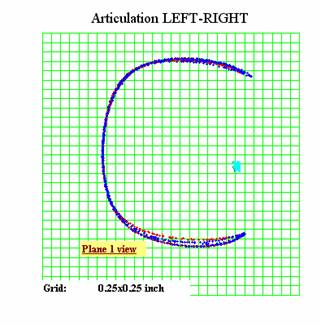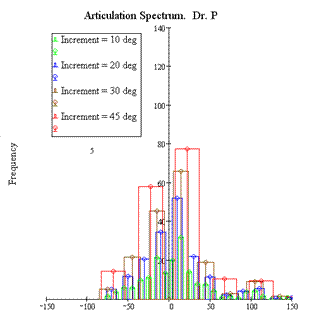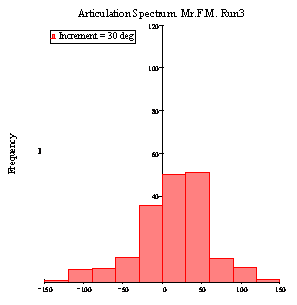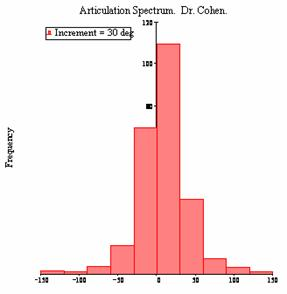Articulation Activity During Colonoscopic Procedure

Written by: R. Tunkel

2005

1.  Executive Summary. 3

2.  Objective. 3

3.  Definitions. 4

4.  Test Setup. 4

6

5.  Test Results. 8

6.  Assumptions and risks of analysis. 13

7.  Conclusions. 14

## 1.    Executive Summary

The purpose of the test described herein was to help understand character, magnitude, and frequency of cycling load for the Articulation Joint (AJ) and its components (pulling wires, plastic tubes, braids, cover, and video cable) during a typical colonoscopy.

The test was designed to measure the total cycling load during an intubation and extraction procedure. A Torso Model with a pig colon was used to conduct this test.

Outcomes include the following:

·        Developed a method for use in upcoming wet lab tests.

·        Obtained articulation spectrums and frequency of completed articulation, which are being used to recommend changes to the SUD specifications.

## 2.Objective

The objective of this report is to describe:

·        the conditions of the lab tests (internal Vet Lab with R&D and Vet Lab #6 with Doctors),

·        the method of collection the articulation related data,

·        the methods and algorithms of data extraction and data analysis, and

·        the possible risks involved in such an analysis based on assumptions of positions of Distal Tip (DT) sensor and Adapter (AD) sensor.

Another objective of this report is to show that such an approach:

·        quantifies the total cycling load (frequency/magnitude) during a colonoscopy, which was applied to a fully packed colonoscope—complete with fluidics tubes, working channel, electrical cables, and four bowden cables with wires and

·        quantifies the different operators’ techniques as a specific articulation spectrum.

The final objective is to draw conclusions about recommendations to the SUD specifications.

## 3.    Definitions

 Term Definition AA articulation angle AD adapter AJ articulation joint CCW counterclockwise CW clockwise DT distal tip extraction procedure the procedure during which the colonoscope is removed from the patient intubation procedure the procedure during which the colonoscope is inserted into the patient LAJ length of articulation joint SUD single use device Torso Model a fixture designed to arrange a pig colon so that it emulates a human colon (see Figure 1)

## 4.Test Setup

### Overview

The following table outlines the testing dates, operators, and equipment:

 Test Date of Test Operator Equipment Internal Vet Lab September 28 Mr. F M Torso Model with Pig Colon (see Figure 1) Vet Lab #6 October 6 Dr. P October 7 Dr. C

Note: The same SUD was used for all tests.Figure 1 shows the torso model with pig colon.

A magnetic tracking system was used to measure the position of Distal Tip (DT) and Articulation Joint (AJ) during intubation and extraction procedures

The two magnetic sensors were attached to the articulation joint during the build process. The distal tip sensor (Sensor 1 in Figure 2) was attached to the distal tip jet wash channel. The adapter sensor (Sensor 2 in Figure 2) was attached to the adapter between the shaft and articulation joint.Figure 2 shows the location of magnetic sensors while calibration is in progress.

### Articulation Algorithm

For the purposes of this test, articulation was defined as motion responsible for change of the distance between two sensors against straight position of AJ. Therefore, a conversion equation was used to calculate the articulation angle (AA) from the known length of the articulation joint (LAJ).

The articulation algorithm (see Figure 3) consisted of the following steps:

1.      Performing the calibration to obtain calibration equation (best fit regression).

2.      Extracting the relevant DT positional data from magnetic tracking.

3.      Checking the positional data for signs of shifting (magnetic sensor discrepancy).

4.      Calculating the LAJ in 3-D space (over time).

5.      Converting the LAJ to AA, using obtained calibration equation.

6.      Offsetting the articulation angle data.

7.      Smoothing the articulation angle data.

8.      Calculating the clockwise and counterclockwise continuous changes in the articulation angle.

9.      Constructing an array of continuous changes in articulation angle without noise around zero (± 5 degrees).

10.  Building the spectrum of AA (+CW, -CCW) with variable increments (10°, 30°, 45°).

11.  Building the spectrum of AA with 45° increment.

12.  Constructing an array of peaks-of-articulation angles (representing completed articulation or final position of DT).

13.  Calculating the number of peaks in ranges: 135°–180° and 90°–135° etc.

14.  Normalizing the number of peaks to standard duration of procedure of 16.6 minutes and worst case procedure time of 32 minutes.Figure 3 shows the articulation calculation algorithm.

### Calibration

The Distance between two magnetic sensors in a straight position of articulation joint (AJ) was approximately 4 inches and was obtained from calibrations (see Figure 4):Figure 4 shows the top view on trajectory of the distal tip magnetic sensor.

The two sensors were not located on the same axis; therefore, these two axes were marginally offset from the axis of rotation of the scope itself. This was clearly seen from the calibration diagrams (Figures 4 and 5)—the articulation trajectories for runs in the 3 different planes (Zero plane, 90-degree plane, and 180-degree plane) were slightly different (±0.125 inch). This results in different LAJ over AA dependences (shown on the right in Figure 5).Figure 5 shows the trajectory of DT in 3-D space and LAJ – AA dependence difference.

The graph on the left in Figure 5 shows the raw data collected, which is represented as a 3D trajectory of the distal tip and adapter sensors. Because of the misalignment of the sensors in the colonoscope, the regression equations for conversion of LAJ to AA were slightly different.

To avoid ambiguity and construct only one equation converting the LAJ to the AA, all three calibration equations (for Zero plane, degree plane and degree plane) were obtained and then averaged (see Figure 6).Figure 6 shows the averaging of the 3 equations for the 3 planes.

Note: Each equation for LAJ (length of articulation joint) over AA (articulation angle) was obtained by averaging 3 runs.

Finally, the calibration equation for Articulation Angle (LAJ) was obtained:## 5.Test Results

During this study, 6 intubations were analyzed—4 during the internal Vet Lab (operator: Mr. M) and 2 during Vet Lab #6 (operators: Dr. P and Dr. C). Mr. F. M also investigated a few extraction procedures.

The articulation calculation was performed for separately for each intubation and each extraction procedure.

For articulation spectrum analysis we define change in articulation as either positive or negative. Positive change in articulation angle is the ascending order over time or clockwise (CW) rotation. Negative change in articulation angle is the descending order over time or counterclockwise (CCW) rotation.

In both cases the Smoothed Articulation Angle function was analyzed. Clockwise articulation was calculated as distances (in degrees) between the minimum and maximum of Articulation Angle, positive in time direction (front angle). Counterclockwise articulation was calculated as distances (in degrees) between the maximum and minimum of Articulation Angle, negative in time direction (back angle).

The Spectrum of articulation was calculated for both clockwise and counterclockwise rotations of Articulation Joint (presented as function with variable increment and without noise around Zero).

The first set of graphs (Figures 7 and 8), which used a variable increment of spectrum (10, 20, 30, and 45 degrees), was designed to better understand the nature of distribution of articulation angles.

The second set of graphs (Figure 9), which used the same increment of spectrum (30 degree), was designed to compare the operators’ behaviors.Figure 7 shows the articulation spectrum (variable increment) for Mr. F. M.

Mr. F. M’s spectrum (Figure 7) shows that he has a smaller number of articulations (when compared to the doctors’ spectrums in Figure 8) progressively increased over trials (from Run1 to Run4). These spectrums are for intubations only. The number of articulations observed during extraction is significantly smaller.Figure 8 shows the articulation spectrums (variable increment) for Dr. P and Dr. C.

Dr. C’s spectrum (Figure 8) shows that he performed much more small articulation in range 20 to 40 degrees that Dr. P.

Dr. C’s spectrum is also more symmetrical (clockwise – counter clockwise) than Dr P’s spectrum.Figure 9 shows the articulation spectrum (30-degree increment) for Mr. F. M.Figure 10 shows the articulation spectrums (30-degree increment) for Dr. P and Dr. C.

A review of the spectrums in Figures 9 and 10 shows that the frequency of cycling load will not exceed 110 cycles for very low magnitude of oscillation (30 degrees).

To quantify this statement statistical analysis was performed and the number of completed articulation (NCA) in a few ranges was obtained. To calculate NCA parameter a maximum of articulation in only one direction (either CW or CCW) was counted (Table 1).

This number represents the frequency of cycling load for the Articulation Joint and its components (pulling wires, plastic tubes, braids, cover, and video cable) during a typical colonoscopy.

Table 1.  Number of completed articulations in four ranges.

 Total Number of Completed Articulation in Range: 1 Run xDr. P315* 1 Run x Dr. C565* 4 Runs xMr. F M140* 400* 400* 210* 5–90 24 55 56 90–135 25 45 7.5 135–160 10 12 4.5 160–180 2 5 2.5

*seconds per run

For comparison, the existing data (Table 1) was extrapolated in Table 2 based on the overall average procedure time and overall worst case procedure time.  Research shows that these are 16.6 minutes and 32 minutes respectively. Only the doctor’s data was used as Mr. F. M was an inexperienced user not trained at colonoscopy.

Table 2.  Extrapolated number of articulations based on Procedure Time

 Articulation in range (Degrees): Dr. P Dr. C Procedure Time (32 minutes Worst Case) (16.6 minutes average procedure) Standard Deviation Average Average + 3  Standard Deviations 5–90 138 176 32 27 157 238 90–135 79.3 79.6 16.6 0.2 79 80 135–160 31.7 21.2 16.6 7 26 49 160–180 6.3 8.8 16.6 2 8 13

The results from Table 2 can be used to set specifications for the articulation durability of the disposable colonoscope to ensure consistent articulation performance for the duration of a worst case procedure (Table 3 and Figure 11).

Longer than average procedures (over 16.6 minutes) are likely to occur for two reasons: 1) because doctors are having difficulty advancing the scope, and/or 2) longer durations performing therapeutic interventions. In both these cases, clinical experience suggests the number of articulations at angles greater than 90 degrees would not increase proportionally with procedure time.  Therefore it is appropriate to model articulations greater than 90 degrees using the average procedure time of 16.6 minutes.

The minimum specification for the 5-90 articulation range is derived from the upper statistical limit of the worst case procedure duration in Table 2. Similarly, the minimum specifications for the remaining three angular ranges (90-135; 135-160; 160-180) are derived from the upper statistical limits of the average procedure time from Table 2, and have been rounded for convenience.

Table 3.  Minimum specification for articulation durability

 Articulation Angle Range Number of completed cycles in range: 5–90 240 90–135 80 135–160 50 160–180 13Figure 11 shows the recommended test numbers.

## 6.    Assumptions and risks of analysis

• Tests were done with unknown (or different) shaft looping configurations.
• Calibration of the magnetic sensors exhibited significant non linearity. As the angle increased the accuracy decreased.
• Anomaly of magnetic field in the Torso Model environment could cause tracking problems.
• A statistically small number of Runs (6) was analyzed.
• Arbitrary noise reduction (± 5o) was performed.
• Arbitrary window size selection for smoothing the collected articulation data.
• Extrapolation of the duration of the procedure to 16.6 minutes and 32 minutes was necessary to make this statistical analysis possible. Because duration of our wet lab tests varied, data was extrapolated by a known average and maximum duration of an endoscopic procedure from a hospital’s study. However, it was done proportionally, which did not reflect the intensity of the procedure.

Therefore, it was believed that this approach correctly represent the reality of colonoscopic procedure in regard of cycling load of the Articulation Joint.

## 7.    Conclusions

1. The observed Level of the cycling of the AJ during the endoscopic procedure did not exceed 5 cycles (for magnitude of cycle in the range 160–180 degrees), 12 cycles (for magnitude of cycle in the range 135–160 degrees) and 45 cycles (for magnitude of cycle in range 90–135 degrees) for 6 performed tests. As for the range 5–90 degrees, the observed level of the cycling of the AJ did not exceed 100 cycles.

2.      The variability of the colon model complexity (changing the shape of the colon within the torso model for each run) made the test very realistic in regards to Human colonoscopy and represented a real life procedure. The differences in the results from run to run were also a result of the variable colon model complexity.

3.      Finding the number of cycles during a procedure could be useful for specifications for durability of the pulling wire because of the resulting wear between the individual wires in the cable and between the wire and springs of the articulation joint.

4.       Finding the magnitude of cycles during a procedure could be useful for specifications for durability of pulling wire, plastic tubes, metal braids, plastic cover, and video cable. All components stretch as the joint is bent. The magnitude of cycles is important because this determines the level of stress in these components.

 Kuznets N. (2002). “Diagnostic colonoscopy: Performance measurement study.” Journal of ambulatory care management, 2002 Jul;25(3):41-55.